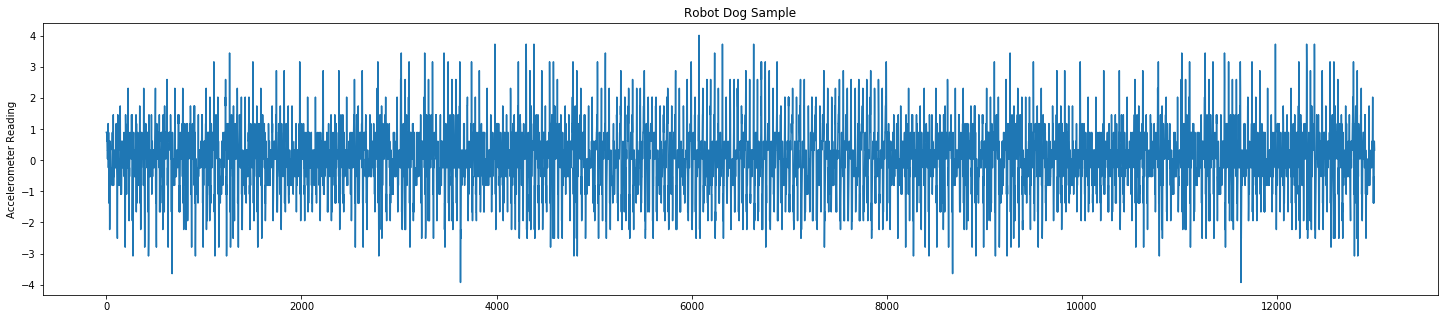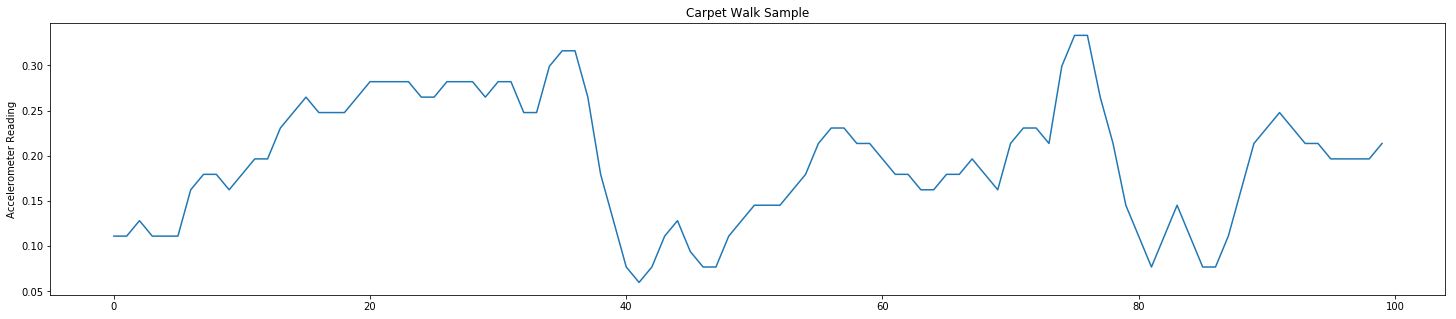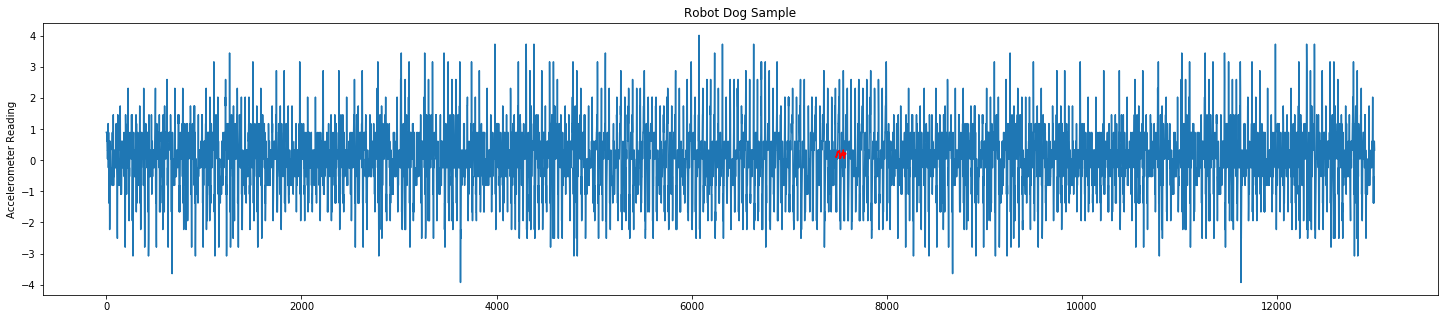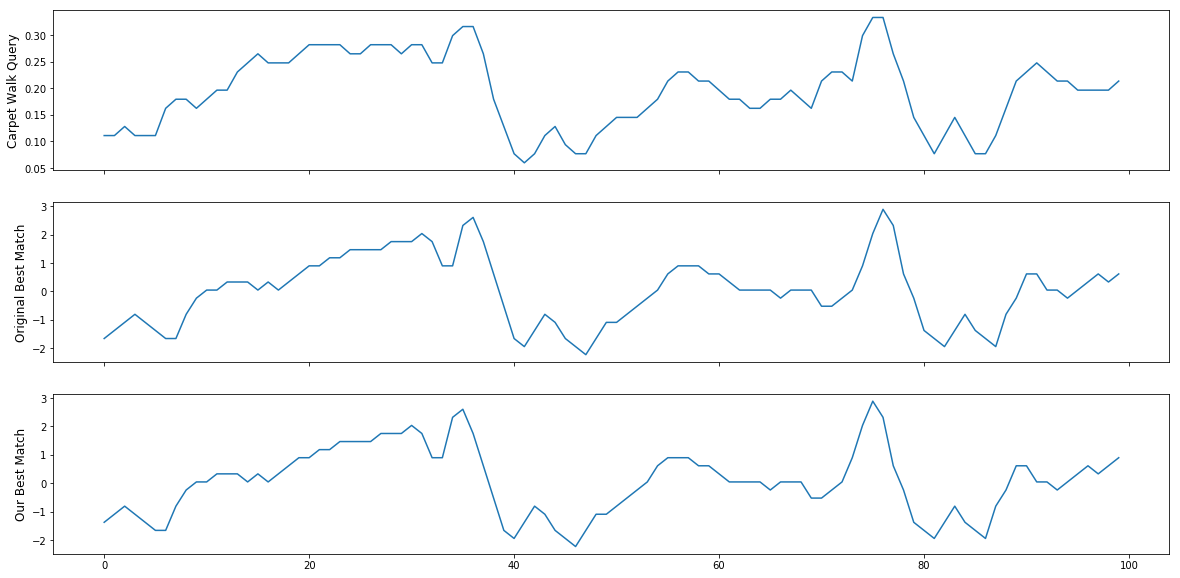# MASS (Mueen's Algorithm for Similarity Search) - Robot Dog Example

This post is used to illustrate how to use mass-ts; a library that I created composed of similarity search algorithms in time series data. For this example, I will walk you through similarity search on the UCR data set - robot dog.

### Data Explaination¶

The dataset comes from an accelerometer inside a Sony AIBO robot dog. The query comes from a period when the dog was walking on carpet, the test data we will search comes from a time the robot walked on cement (for 5000 data points), then carpet (for 3000 data points), then back onto cement.

Note
All of the code in this example is using Python 3.

In :
import numpy as np
import mass_ts as mts
from matplotlib import pyplot as plt

%matplotlib inline


In :
robot_dog = np.loadtxt('robot_dog.txt')


### Plot Data¶

In :
plt.figure(figsize=(25,5))
plt.plot(np.arange(len(robot_dog)), robot_dog)
plt.title('Robot Dog Sample')
plt.show()The series data for the robot dog is difficult to see a pattern unless you make the plot very large. At 25 by 5 you can see a pattern in the middle of the data set. This is where the carpet walk takes place.

In :
plt.figure(figsize=(25,5))
plt.plot(np.arange(len(carpet_walk)), carpet_walk)
plt.title('Carpet Walk Sample')
plt.show()## Search for Carpet Walk¶

Now we can search for the carpet walk snippet within the robot dog sample using MASS.

In :
distances = mts.mass3(robot_dog, carpet_walk, 256)

In :
min_idx = np.argmin(distances)

In :
min_idx

Out:
7479

The minimum index found is the same as the author claims. We can now visualize this below.

In :
plt.figure(figsize=(25,5))
plt.plot(np.arange(len(robot_dog)), robot_dog)
plt.plot(np.arange(min_idx, min_idx + 100), carpet_walk, c='r')
plt.title('Robot Dog Sample')
plt.show()In :
# Plot the carpet walk query
fig, (ax1, ax2, ax3) = plt.subplots(3,1,sharex=True,figsize=(20,10))
ax1.plot(np.arange(len(carpet_walk)), carpet_walk)
ax1.set_ylabel('Carpet Walk Query', size=12)

# Plot use case best match from original authors
ax2.plot(np.arange(100), robot_dog[7478:7478+100])
ax2.set_ylabel('Original Best Match', size=12)

# Plot our best match
ax3.plot(np.arange(100), robot_dog[min_idx:min_idx+100])
ax3.set_ylabel('Our Best Match', size=12)

plt.show()Contents © 2020 Tyler Marrs